# 2.6. PARALLEL LANGUAGES

## 2.5. ARRAY LIBRARIES

As we have seen, the most difficult problem to be solved by optimizing C and Fortran 77 compilers is the recognition of the loops, which can be parallelized or vectorized. Function extensions of C and Fortran 77 with array or vector libraries allow the programmer to use regular C and Fortran 77 compilers, which perform no optimizations specific for vector and superscalar architectures, for efficient programming those architectures. Instead, the programmer can use library functions or subroutines efficiently implementing operations on arrays for each particular vector or superscalar processor.

One well-known and well-designed array library is the Basic Linear Algebra Subprogram (BLAS). This library provides basic array operations for numerical linear algebra, and it is aimed, first of all, at vector and superscalar architectures. The functionality of the library covers all main operations on vectors and matrices used for the numerical solution of linear algebra problems.

Highly efficient machine-specific implementations of the BLAS are available for most modern computers, and this is what makes parallel programming with the library efficiently portable.

BLAS routines are divided into three main categories, commonly referred to as Levels 1, 2, and 3 BLAS, depending on the type of implemented operation. Level 1 addresses scalar-vector operations, Level 2 addresses matrix-vector operations, and Level 3 addresses matrix-matrix operations.

### 2.5.1. Level 1 BLAS

Routines of Level 1 do vector reduction operations, vector rotation operations, elementwise and combined vector operations, and data movement with vectors. An example of vector reduction operation is the addition of the scaled dot product of two real vectors x and y into a scaled scalar r. The mathematical formulation of the operation is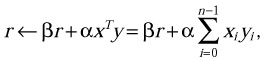where α, β, r Є , x, y Є n, xT denotes the transposition of vector x, and stands for the assignment statement. The BLAS provides both Fortran 77 and C interfaces to its routines. The C interface of the routine implementing the above operation is

` void BLAS_ddot( enum blas_conj_type conj, int n,                 double alpha, const double *x,                  int incx, double beta, const double *y,                  int incy, double *r );`

The n-length vector operand x is specified by two arguments—x and incx, where x is an array that contains the entries of the n-length vector x, and incx is the stride within x between two successive elements of the vector x. The argument conj has no effect and is only added to keep interfaces of different routines but implementing similar operations generic. This optional argument specifies for the function BLAS_cdot, which implements the same operation but on complex vectors x and y, if the vector components xi are used unconjugated or conjugated. Other routines of the group compute different vector norms of vector x, compute the sum of the entries of vector x, find the smallest or biggest component of vector x, computes the sum of squares of the entries of vector x. Level 1 routines doing rotation operations generate Givens plane rotation, Jacobi rotation, and Householder transformation.

An example of elementwise vector operation is the scaled addition of two real vectors x and y. The mathematical formulation of the operation is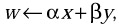where α, β Є , and x, y, w Є n. The C interface of the routine implementing this operation is

` void BLAS_dwaxpby( int n, double alpha, const double *x,                    int incx, double beta, const double *y,                    int incy, double *w, int incw );`

The routine scales vector x by α and vector y by β, adds these two vectors, and stores the result in vector w. Function BLAS_cwaxpby does the same operation but on complex vectors. Other routines of the group scale the entries of a vector x by the real scalar 1/α; scale a vector x by α and a vector y by β, add these two vectors to one another, and store the result in vector y; combine a scaled vector accumulation and a dot product; and apply a plane rotation to vectors x and y.

An example of data movement with vectors is the interchange of real vectors x and y. The C interface of the routine implementing this operation is

` void BLAS_dswap( int n, double *x, int incx, double *y,                  int incy );`

Function BLAS_cswap does the same operation but on complex vectors. Other routines of the group copy vector x into vector y; sort the entries of real vector x in increasing or decreasing order, and overwrite this vector x with the sorted vector as well as compute the corresponding permutation vector p; permute the entries of vector x according to permutation vector p.

### 2.5.2. Level 2 BLAS

Routines of Level 2 compute different matrix vector products, do addition of scaled matrix vector products, compute multiple matrix vector products, solve triangular equations, and perform rank 1 and rank 2 updates. Matrix operands of the operations are dense or banded. In addition some operations use symmetric, Hermitian, or triangular matrices. To store matrices, the following schemes are used:

• Column-based and row-based storage in a contiguous way (conventional storage).

• Packed storage for symmetric, Hermitian or triangular matrices.

• Band storage for band matrices.

So, for the C language interfaces, matrices may be stored in columns or rows: an m X n matrix A is stored in a one-dimensional array a, with matrix element aij stored columnwize in an array element a[i+j*s] or row-wize in an array element a[j+i*s]. That is, it is assumed that for row-wize storage, elements within a row are contiguous in memory, while elements within a column are separated by s memory elements. If s is equal to n, the rows will be contiguous in memory. If s is greater that n, there will be a gap of (s - n) memory elements between two successive rows. Similarly, for column-wize storage, elements within a column are contiguous in memory, while elements within the rows are separated by s memory elements. If s is equal to m, rows will be contiguous in memory. If s is greater that m, there will be a gap of (s - n) memory elements between two successive rows.

If a matrix is triangular, only the elements of the upper or lower triangle are accessed. The remaining elements of the array need not be set. Routines that handle symmetric or Hermitian matrices allow for either the upper or lower triangle of the matrix to be stored in the corresponding elements of the array; the remaining elements of the array need not be set.

Symmetric, Hermitian, or triangular matrices are allowed to be stored more compactly, with the relevant triangle being packed by columns or rows in a one-dimensional array. For example, in the case of the C language and row storage scheme, the upper triangle of an n X n matrix A may be stored in a one-dimensional array a in such a way, that matrix element aij (i j) is stored in array element a[j+i*(2*n-i-1)/2]. Thus matrixmay be stored compactly in a one-dimensional array as follows:Compared to general matrices, band matrices are allowed to be stored more compactly. For example, in Fortran and the column-wize storage scheme, an m X n band matrix A with l subdiagonals and u superdiagonals may be stored in a two-dimensional array A with l + u + 1 rows and n columns. Columns of matrix A are stored in corresponding columns of array A, and diagonals of matrix A are stored in rows of array A in such a way that matrix element aij is stored in array element A(u+i-j,j) for max(0, j - u) ≤ i ≤ min(m - 1, j + l). Thus band matrix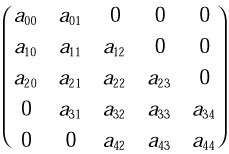may be stored compactly in a two-dimensional array as follows: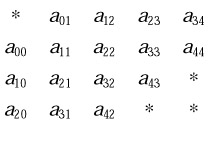The elements marked by an * in the upper left and lower right corners of this array need not be set because they are not referenced by BLAS routines.

There are also special storage schemes for compact storing triangular, symmetric, and Hermitian band matrices. An example of matrix vector multiplication operation is the scaled addition of a real n-length vector y, and the product of a general real m X n matrix A and a real n-length vector x. The mathematical formulation of the operation is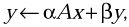where α, β Є_. The C interface of the routine implementing this operation is

` void BLAS_dgemv( enum blas_order_type order,                  enum blas_trans_type trans, int m, int n,                  double alpha, const double *a, int stride,                  const double *x, int incx, double beta,                  const double *y, int incy );`

If order is set to blas_rowmajor, it is assumed that the matrix A is stored row-wise in the array a with elements within array columns separated by stride memory elements. If this parameter is set to blas_colmajor, it is assumed that A is stored columnwise with elements within array rows separated by stride memory elements. The argument trans should be set to blas_no_trans. If the parameter is set to blas_trans, the routine will perform the operation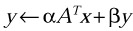instead of the operation above. Function BLAS_cgemv performs the same operation but on complex operands. If matrix A is a general band matrix with l subdiagonals and u superdiagonals, the use of the function

` void BLAS_dgbmv( enum blas_order_type order,                  enum blas_trans_type trans,                  int m, int n, int l, int u,                  double alpha, const double *a, int stride,                  const double *x, int incx, double beta,                  const double *y, int incy );`

instead of BLAS_dgemv leads to better use of memory. Function BLAS_dgbmv assumes that a band storage scheme is used to store matrix A.

Other routines of Level 2 do the following:

• Multiply vector x by a real symmetric, complex symmetric, or Hermitian matrix A; scale the resulting vector and add it to the scaled vector operand y, that is, perform the operationwhere A = AT or A = AH.

• Multiply vector x by a general triangular matrix A, or its transpose, or its conjugate transpose, and copy the resulting vector in the vector operand x, that is, perform one of the operations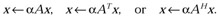• Add the product of two scaled matrix vector products, that is, perform the operation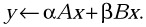• Multiply vector y by a general matrix AT, scale the resulting vector, and add the result to z, storing the result in the vector operand x; then multiply the matrix A by x, scale the resulting vector, and store it in the vector operand w; that is, perform the pair of operations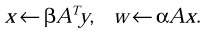• Multiply vector y by a triangular matrix AT, storing the result as x, as well as multiply matrix A by vector z, storing the result as w; that is, perform the pair of operations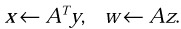• Precede a combined matrix vector and a transposed matrix vector mul-_tiply by a rank 2 update—namely update a matrix A by u1v1T and u2v2T, then multiply the updated matrix by a vector y, then add the scaled resulting vector to the vector operand z, storing the result as x, then multiply the operand x by the updated matrix A, and then scale the resulting vector and store it as w; that is, perform the sequence of operations• Solve one of the systems of equations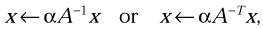where x is a vector and matrix A is a unit, non-unit, upper or lower triangular (or triangular banded) matrix.

• Perform the rank 1 operation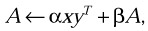where x is a vector and A is a unit, non-unit, upper or lower triangular (or triangular banded) matrix.

• Perform the symmetric rank 1 update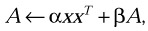where α and β are scalars, x is a vector, and A is a symmetric matrix.

• Perform the Hermitian rank 1 update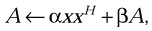where α and β are real scalars, x is a complex vector, and A is a Hermitian matrix.

• Perform the symmetric rank 2 update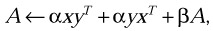where α and β are scalars, x and y are vectors, and A is a symmetric matrix.

• Perform the Hermitian rank 2 update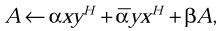where α is a complex scalar and β is a real scalar, x and y are complex vectors, and A is a Hermitian matrix.

For any matrix-vector operation that has a specific matrix operand (triangular, symmetric, banded, etc.), there is a routine implementing the operation for each storage scheme that can be used to store the operand.

### 2.5.3. Level 3 BLAS

Routines of Level 3 do O(n2) matrix operations (norms, diagonal scaling, scaled accumulation, and addition), O(n3) matrix-matrix operations (multiplication, solving matrix equations, symmetric rank k and 2k updates), and data movement with matrices.

Routines implementing O(n2) matrix operations allow the programmers to use different storage schemes to store matrix operands. Depending on the form of the matrix operands, different conventional, packed, or band storage schemes can be used. For example, to add two symmetric band matrices, we can pick a routine using the general conventional storage scheme, a routine using the band storage scheme, a routing using the conventional symmetric storage scheme, a routine using the symmetric band storage scheme, or a routine using the symmetric packed storage scheme.

An example of the O(n2) matrix operation is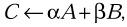which scales two real m X n matrices A and B and stores their sum in a matrix C. The C interface of the routine implementing this operation under assumption that the matrices A, B, and C are of the general form is

` void BLAS_dge_add( enum blas_order_type order, int m,                          int n, double alpha, const double *a,                         int stride_a, double beta,                          const double *b, int stride_b,                          double *c, int stride_c );`

There are other 15 routines performing this operation for different types and forms of the matrices A, B, and C. For example, function BLAS_cge_add performs this operation on complex matrices of the general form, and function BLAS_dtp_add performs this operation on real triangular matrices, which are stored using the packed storage scheme.

An example of O(n3) matrix-matrix operation is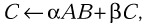where α, β are scalars, A is an m X n matrix, B is an n X k matrix, and C is an m X k matrix. The C interface of the routine implementing this operation under assumption that A, B and C are real matrices of the general form is

` void BLAS_dgemm( enum blas_order_type order,       enum blas_trans_type trans_a,       enum blas_trans_type trans_b,       int m, int n, int k, double alpha,       const double *a, int stride_a,       const double *b, int stride_b,       double beta, const double *c, _      int stride_c ) ;`

Arguments trans_a and trans_b should be set to blas_no_trans. If, say, the parameter trans_a is set to blas_trans, the routine will perform the operation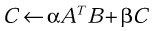instead of the operation above. Function BLAS_cgemm performs the same operation but on complex operands. Other routines of the group do the following:

• Perform one of the symmetric (or Hermitian) matrix-matrix operations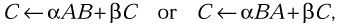where α and β are scalars, A is a symmetric (or Hermitian) matrix, and B and C are general matrices.

• Perform one of the matrix-matrix operations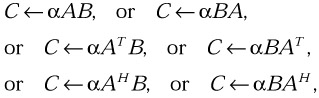where α is a scalar, B is a general matrix, and A is a triangular (or triangular band) matrix.

• Solve one of the matrix equations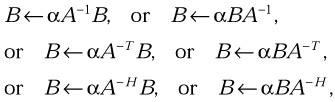where α is a scalar, B is a general matrix, and A is a triangular matrix.

• Perform one of the symmetric rank k and 2k updates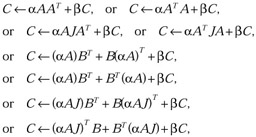where α is a scalar, C is a symmetric matrix, A and B are general matrices, and J is a symmetric tridiagonal matrix.

• Perform one of the Hermitian rank k and 2k updates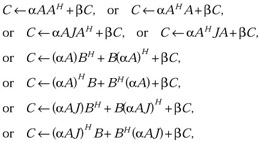where α is a scalar, C is a Hermitian matrix, A and B are general matrices, and J is a symmetric tridiagonal matrix.

Data movement with matrices include

• copying matrix A (or its transpose or conjugate transpose), with storing the result in matrix B,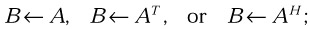• transposition or conjugate-transposition of a square matrix A with the result overwriting matrix A,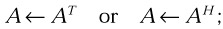• permutation of the rows or columns of matrix A by a permutation matrix P,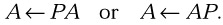Routines implementing the operations support different types and forms of matrix operands as well as different storage schemes.

### 2.5.4. Sparse BLAS

Apart from the Dense and Banded BLAS outlined above, there is a library called the Sparse BLAS providing computational routines for unstructured sparse matrices, that is, such matrices that contain many zero entries not making up a regular sparsity pattern (e.g., banded or triangular).

The Sparse BLAS functionality is much poorer than that of the Dense and Banded BLAS and only includes matrix multiply and triangular solve, along with sparse vector update, dot product, and gather/scatter (the basic array operations used in solving large sparse linear equations by iterative techniques).

The Sparse BLAS does not specify a method to store the sparse matrix. The storage format is dependent on the algorithm being used, the original sparsity pattern of the matrix, the underlying computer architecture, among other considerations about the format in which the data exists, and so on. Therefore sparse matrix arguments to the Sparse BLAS routines are not really data components but placeholders, or handles, that refer to an abstract representation of the matrix. Several routines are provided to create Sparse BLAS matrices, but the internal representation is implementation dependent, which provides Sparse BLAS library developers with the best opportunity for optimizing and fine-tuning their kernels for specific situations. As a result programmers can write numerical algorithms using the Sparse BLAS independently of the matrix storage scheme, relying on the scheme provided by each particular implementation of the library.

The typical use of the Spase BLAS consists of three phases:

• Create an internal sparse matrix representation and return its handle.

• Use the handle as a parameter in computational Sparse BLAS routines.

• Call a cleanup routine to free resourses associated with the handle, when the matrix is no longer needed.

The C program below illustrates the use of the Sparse BLAS library to multiply a sparse 4 X 4 matrix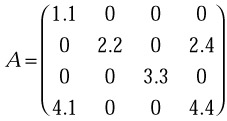with the vector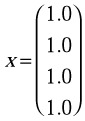performing the operation y Ax:

`#include <blas_sparse.h> int main() {       const int n = 4;       const int nonzeros = 6;       double values[] = {1.1, 2.2, 2.4, 3.3, 4.1, 4.4};       int index_i[] = {0, 1, 1, 2, 3, 3};       int index_j[] = {0, 1, 3, 2, 0, 3};       double x[] = {1.0, 1.0, 1.0, 1.0};       double y[] = {0.0, 0.0, 0.0, 0.0};       blas_sparse_matrix A;       int k;       double alpha = 1.0;       /* Create Sparse BLAS handle */       A = BLAS_duscr_begin(n, n);       /* Insert entries one by one */       for(k=0; k < nonzeros; k++)             BLAS_duscr_insert_entry(A, values[k], index_i[k],              index_j[k]);       /* Complete construction of sparse matrix */       BLAS_uscr_end(A);       /* Compute matrix-vector product y = A*x */       BLAS_dusmv(blas_no_trans, alpha, A, x, 1, y, 1);       /* Release matrix handle */       BLAS_usds(A); }`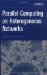Parallel Computing on Heterogeneous Networks (Wiley Series on Parallel and Distributed Computing)
ISBN: B000W7ZQBO
EAN: N/A
Year: 2005
Pages: 95